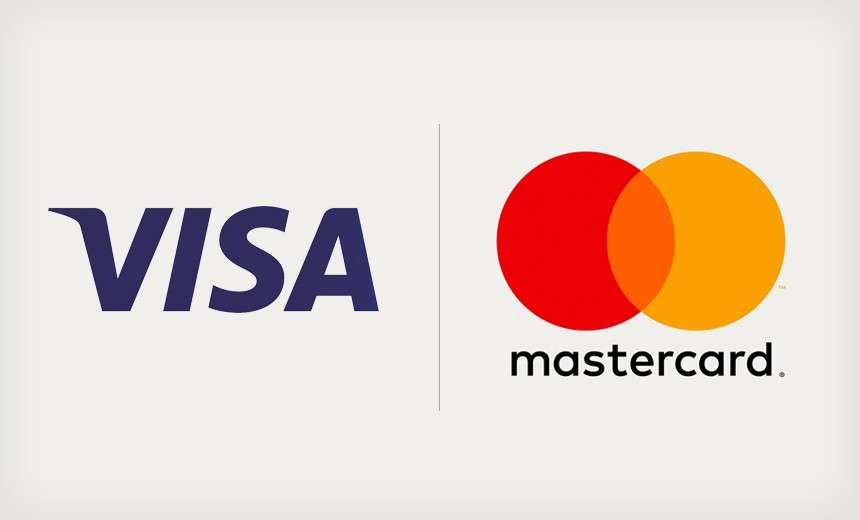# Transparent High Heel Pumps

Regular price
R 999.00
Sale price
R 999.00
Quantity must be 1 or more

These transparent high heels are the hottest thing at the moment. The pictures speak for themselves. They feature an 12 cm heel. Order now.

Use the below size table for selecting a size.

size 4=35=feet length 22.5CM
size 5=36=feet length 23CM
size 6=37=feet length 23.5CM
size 7=38=feet length 24CM
size 8=39=feet length 24.5CM
size 9=40=feet length 25CM
size 10=41=feet length 25.5CM
size 11=42=feet length 26CM

Heels height: 12cm
Platform height: 1cm